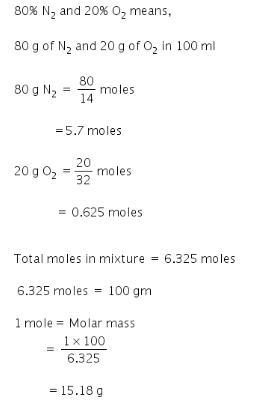# A sample contain 80% of n2 and 20% mole of O2. find molar mass

A sample contain 80% of n2 and 20% mole of O2. find molar mass

Given:
N2 = 80%
O2 = 20%
Molar mass=?Molar mass is 15.18